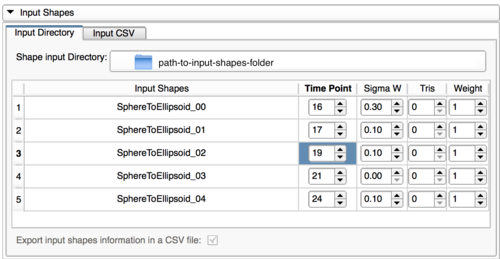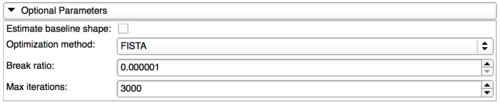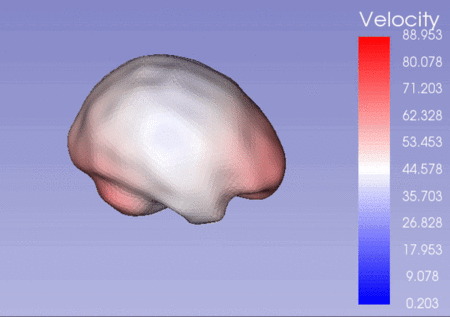# Difference between revisions of "Documentation/Nightly/Extensions/ShapeRegressionExtension"

Home < Documentation < Nightly < Extensions < ShapeRegressionExtension
 For the stable Slicer documentation, visit the 4.10 page.

# Introduction and Acknowledgements

 Extensions: ShapeRegressionExtension Author: Laura Pascal (Kitware Inc.) Contributors: Beatriz Paniagua (Kitware Inc), James Fishbaugh (University of North Carolina) Contact: Laura Pascal, laura.pascal@kitware.com, Beatriz Paniagua, beatriz.paniagua@kitware.com Acknowledgments: NIH NIBIB R01EB021391 (Shape Analysis Toolbox for Medical Image Computing Projects) License: Apache License, Version 2.0

# Extension Description

This extension is composed by two modules :

• RegressionComputation:
• Computation of time-regressed shapes in a collection of 3D shape inputs associated to a linear variable thanks to shape4D CLI.
• RegressionVisualization:
• Plotting of the time-regressed shape volume evolution according to a linear variable
• Visualization of the sequence of the time-regressed shapes generated (using Sequences module)

# Interface

Regression Computation module:

Regression Visualization module:

# Use Cases

## Regression Computation Module

This module is a user-friendly interface allowing to easily use shape4D CLI in order to compute time-regressed shapes in a collection of 3D shape inputs associated to a linear variable.

### Shape4D InputsRegression Computation Input Shape Parameters This section allows the user to specify the input shapes for each time point as well as their associated parameters. To do so, the user can either specify : A folder containing the input shapes. The user would then have to specify the parameters associated to each input shape. A CSV file (CSVInputshapeparameters.csv) containing all these information will be automatically created in the output folder specified by the user. A CSV files containing in each row the input shape file path followed by the shape parameters "Time Point" , "Sigma W" , "Tris" and "Weight" .Example of Regression Computation Input CSV file

### Shape4D Parameters

#### Time ParametersRegression Computation Time Parameters This section allows the user to specify: The first and last time points of the input shapes The number of time-regressed shapes that will be computed between the first and last input shapes

#### Output Parameters

Shape4D CLI will compute several sets of time-regressed shapes where the basename of each shape will be: <prefix>_iter_n_time_00i with:
• <prefix>: the prefix can be specified by the user
• n: the iteration number of the shape regression process (i.e. represents the number of the time-regressed shape serie)
• i: the time-regressed shape number
The last iteration will create the final time-regressed shape set where the basename of the shapes will be <prefix>_final_time_00i.Regression Computation Output Parameters This section allows the user to specify : The output folder where the computed time-regressed shape sets will be stored The prefix of the basename of each time-regressed shapes The occurrence at which the time-regressed shapes set will be saved

#### Optimal ParametersRegression Computation Optimal Parameters This section allows the user to specify: The optimal method used by Shape4D CLI The minimum distance that will stopped the shape regression process The maximum number of iteration

## Regression Visualization Module

This module allows to easily visualize the results obtained by the RegressionComputation module by using severals slicer extensions (Sequences, Colors, Tables).

### Inputs

This section allows the user to create a sequence containing the time-regressed shapes computed by RegressionComputation module.
 In order to do that, the user would have to specify: The input directory which should contain the time-regressed shape sets computed by RegressionComputation module The rootname of the time-regressed shape set that should be _iter_n_time_ with: The prefix specified by the user in the RegressionComputation module n the iteration number of the shape regression process that the user want to visualize (iter_n will be replaced by final if the user wants to display the final results computed)

### Visualization

#### Sequence Visualization

 In this tab, the user could: customize the color maps of the shapes contained in the sequence navigate through the sequence thanks to a browserExample of a brain growth sequence

#### Regression Plot

This tab allows the user to plot the time-regressed shape volume evolution according to the time.
 To do so, the user will have to specify the path of a CSV file containing the following information used to compute the set of time-regressed shapes in RegressionComputation module: The path of each input shape The time-point associated